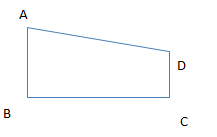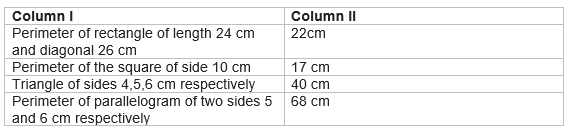# Heron's formula class 9 extra questions

Given below are the Class 9 Maths Important Questions and Extra Questions for Heron's Formula
(a) Concepts questions
(b) Calculation problems
(c) Multiple choice questions
(e) Fill in the blank's

## 1 Mark Questions

Question 1
Calculate the area in each case
1. Triangle have sides as a=5 cm ,b=4 cm,c=3 cm
2. Equilateral triangle having side a=2 cm
3. Right angle triangle have base=4 cm and Height =3 cm
4. Square whose diagonal is 10 cm
5. Rectangle whose length and breath are 6 and 4 cm
6. Parallelogram whose two sides are 10 cm and 16 cm and diagonal is 14 cm
7. Parallelogram whose base is 10 cm and height is 14 cm
8. Rhombus of diagonals to 10 and 24 cm
9. Two sides of trapezium are 36 and 24 cm and its altitude is 12 cm.
Solution
(a) $s=\frac{a+b+c}{2}$ =6
Area
$A=\sqrt{s(s-a)(s-b)(s-c)}$=6cm2
(b) Area of equilateral
$A=\frac{\sqrt{3}}{4}a^{2}=\sqrt{3}$
(c) Area of triangle
A=(1/2)BH=6cm2
(d) Area of square in terms diagonal
A=(1/2)d2=50cm2
(e) Rectangle area is given by
A=LXB=24cm2
(f) In parallelogram whose two sides and diagonal are given, Area is given by
$A=\sqrt{s(s-a)(s-b)(s-d)}$
Where $s=\frac{a+b+d}{2}$
So s=20cm
So A=80(3)1/2cm2
(g) Area is given by
A=Base X height =10X14=140cm2
(h) Area is given by
A=(1/2)d1d2=120cm2
(i) Area of trapezium is given by
A=(1/2)(Sum of parallel sides) Altitude
A=360cm2

## True and False

Question 2
True or False statement
(a) Heron formula for area of triangle is not valid of all triangles
(b) If each side of the triangles is tripled, the area will becomes 9 times
(c) Base and corresponding altitude of the parallelogram are 8 and 5 cm respectively.Area of parallelogram is 40 cm2
(d) If each side of triangle is doubled, the perimeter will become 4 times
(e) If p is the perimeter of the triangle of sides a,b,c ,the area of triangle is
$A=\frac{1}{4}\sqrt{p(p-2a)(p-2b)(p-2c)}$
(f) When two triangles are congruent, there areas are same
(g) Heron’s belongs to America
(h) If the side of the equilateral triangle is a rational number, the area would always be irrational number
Solution
1. False
2. True
3. True
4. False
5. True
6. True
7. False
8. True

## Multiple choice Questions

Question 3
The difference between sides at right angles in a right angled triangle is 14 cm. The area of the triangle is 120 cm2 . The perimeter of the triangle is
(a) 80
(b) 45
(c) 60
(d) 64
Solution
(c)
Let y be one of the at right angle ,then another side will be y-14
Now we know that
A=(1/2)BH
120=(1/2)y(y-14)
y2-14y-240
(y-24)(y+10)=0
y=24
So other side is 10
From pythogrous theorem
$\text{hyp}=\sqrt{10^{2}+24^{2}}$=26cm
So perimeter will be =10+24+26=60 cm

Question 4
Find the area of the equilateral triangle whose perimeter is 180 cm?
Which of the following is true?
(a)900(3)1/2 cm2
(b)300(3)1/2 cm2
(c)200(3)1/2 cm2
(d) None of these
Solution
P=3a => a=P/3=60 cm
Area of equilateral
$A=\frac{\sqrt{3}}{4}a^{2}$

Question 5
One diagonal and side of the rhombus are 24 and 13 cm respectively, Find the Area and other diagonal size?
(a) 160 cm2,10 cm
(b) 120 cm2,20 cm
(c) 120 cm2,10 cm
(d) None of the above
Solution

Question 6
ABCD is a trapezium with AB =10cm, AD=5 cm, BC=4 cm and DC =7 cm?Find the area of the ABCD
(a) 34 cm2
(b) 28cm2
(c) 20 cm2
(d) None of these
Solution a
BC is the altitude between the two parallel sides AB and DC
So Area of trapezium will be given by
$A=\frac{1}{2}BC(AB+DC)$=34cm2

Question 7
Find the area and perimeter of the right angle triangle whose hypotenuse is 5 cm and Base is 4 cm
(a) 6 cm2 ,12 cm
(b) 12 cm2 ,14 cm
(c) 4 cm2, 6 cm
(d) 12 cm2 ,6 cm
Solution
By pythogorous theorem
$height=\sqrt{hyp^{2}-base^{2}}=\sqrt{25-19}=3$
So Area =(1/2) XBase X height=6 cm2
Perimeter = 5+4+3=12 cm

Question 8
In an isosceles triangle ?ABC with AB = AC=13 cm. D is mid point on BC. Also BC=10 cm
Which of the following is true?
(a) Area of Triangle ABD and ADC are equal
(b) Area of triangle ABD is 30 cm2
(c) Area of triangle ABC is 60 cm2
(d) All the above
Solution
ABD an ADC are congruent triangle, So Area of Triangle ABD and ADC are equal
Also From pythogorous theorem, AD will be given as
$AD=\sqrt{AB^{2}-BD^{2}}=\sqrt{169-25}=12cm$
So Area of triangle ABC=(1/2)X base X height=60 cm2

Question 9
A triangle and a parallelogram have the same base and the same area. The sides of the triangle are 26 cm and 30 cm and parallelogram stands on the base 28 cm. calculate the height of the parallelogram
(a) 12 cm
(b) 14 cm
(c) 10cm
(d) 13 cm
Solution
For triangle, all the sides are given, calculating the area using Heron formula
A=336 cm2
Now for parallelogram, Area is given by
A= Base X Altitude
336=28 X H
Or H=12 cm

Question 10
Find the percentage increase in size of the triangle if each side is doubled
(a)
(b) 300%
(c) 400%
(d) None of the above
Solution

## Match the column

Question 11Question 12
If each side of a triangle is doubled, then find the ratio of area of the new triangle thus formed and the given triangle
Solution
Let $s=\frac{a+b+c}{2}$
Area
$A=\sqrt{s(s-a)(s-b)(s-c)}$ Now when each side of the traingle is doubled Sides will be 2a, 2b,2c Then $s_f=\frac{2a+2b+2c}{2}=2s$ $A_f=\sqrt{2s(2s-2a)(2s-2b)(2s-2c)}= 4 \sqrt{s(s-a)(s-b)(s-c)}=4A$
Question 13
The perimeter of a triangle is 50 cm. One side of a triangle is 4 cm longer than the smaller side and the third side is 6 cm less than twice the smaller side. Find the area of the triangle.
Solution
let x be the smaller side,then other sides are x+4 , 2x-6
Now x + x+4+ 2x-6=50
4x=52
x=13
So sides are 13,17, 20
Now s=25 cm
$A=\sqrt{s(s-a)(s-b)(s-c)}= \sqrt {25(25-13)(25-17)(25-20)}=\sqrt {25 \times 12 \times 8 \times 5}=20 \sqrt {30} cm^2$

Question 14
The area of a trapezium is 475 cm2 and the height is 19 cm. Find the lengths of its two parallel sides if one side is 4 cm greater than the other.
Solution
let x be one parallel side,then other is x+4
Now
$475= \frac {1}{2} 19 (x+ x+4)$
x=23
So two parallel sides are 23 and 27 cm

## Summary

This Heron's formula class 9 extra questions with answers is prepared keeping in mind the latest syllabus of CBSE . This has been designed in a way to improve the academic performance of the students. If you find mistakes , please do provide the feedback on the mail.